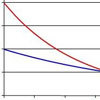#### You may also like### Spinners

How do scores on dice and factors of polynomials relate to each other?### Data Matching

Use your skill and judgement to match the sets of random data.### Into the Exponential Distribution

Get into the exponential distribution through an exploration of its pdf.

# Random Inequalities

##### Age 16 to 18Challenge Level

In this problem we look at two general 'random inequalities'.

Part 1
Markov's inequality tells us that the probability that the modulus of a random variable $X$ exceeds any random positive number $a$ is given by a universal inequality as follows:

$$P(|X|\geq a) \leq \frac{E(|X|)}{a^{??}}$$
In this expression the exponent of the denominator on the right hand side is missing, although Markov showed that it is the same whole number for every possible distribution. Given this fact, experiment with the various distributions to find the missing value (??).

Part 2
Another important general statistical result is Chebyshev's inequality, which says that

$$P(|X-\mu|\geq k\sigma)\leq \frac{1}{k^2}$$ where $\mu$ and $\sigma$ are the mean and standard devitation of the distribution $X$ respectively. This is true for any distribution and any positive number $k$. Can you make a probability distribution for which the inequality is exactly met when $k=2$? In other words, use the distribution maker to create a distribution $X$ for which $$P(|X-\mu|\geq 2\sigma)=\frac{1}{4}$$